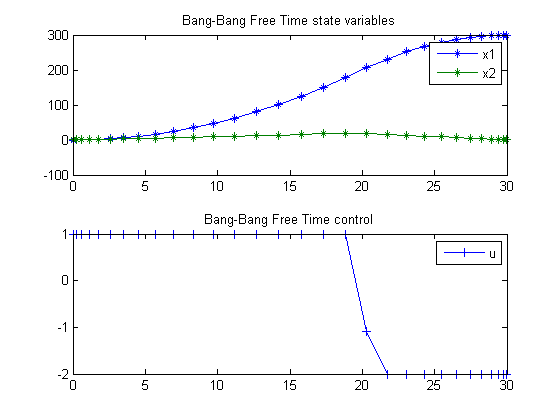10  A Linear Problem with Bang Bang Control

Paper: Solving Tough Optimal Control Problems by Network Enabled Optimization Server (NEOS)

Jinsong Liang, YangQuan Chen, Max Q.-H. Meng, Rees Fullmer Utah State University and Chinese University of Hong Kong (Meng)

EXAMPLE-1: A TEXTBOOK BANG-BANG OPTIMAL CONTROL PROBLEM

10.1  Problem description

Find u over t in [0; t_F ] to minimize

 J = tF

subject to:

 dx1 dt
= x2
 dx2 dt
= u

 x1(0) = 0
 x1(tF) = 300
 x2(0) = 0
 x2(tF) = 0

 −2 <= u <= 1

Reference: 

10.2  Problem setup

toms t
toms t_f

p = tomPhase('p', t, 0, t_f, 30);
setPhase(p);

tomStates x1 x2
tomControls u

% Initial guess
% Note: The guess for t_f must appear in the list before expression involving t.
x0 = {t_f == 20
icollocate({x1 == 300*t/t_f; x2 == 0})
collocate(u==1-2*t/t_f)};

% Box constraints
cbox = {10 <= t_f <= 40
-2 <= collocate(u) <= 1};

% Boundary constraints
cbnd = {initial({x1 == 0; x2 == 0})
final({x1 == 300; x2 == 0})};

% ODEs and path constraints
ceq = collocate({dot(x1) == x2; dot(x2) == u});

% Objective
objective = t_f;

10.3  Solve the problem

options = struct;
options.name = 'Bang-Bang Free Time';
options.prilev = 1;
solution = ezsolve(objective, {cbox, cbnd, ceq}, x0, options);
t  = subs(collocate(t),solution);
x1 = subs(collocate(x1),solution);
x2 = subs(collocate(x2),solution);
u  = subs(collocate(u),solution);
Problem type appears to be: lpcon
Starting numeric solver
===== * * * =================================================================== * * *
TOMLAB - Tomlab Optimization Inc. Development license  999001. Valid to 2011-02-05
=====================================================================================
Problem: ---  1: Bang-Bang Free Time            f_k      30.019823270451944000
sum(|constr|)      0.000028878808460443
f(x_k) + sum(|constr|)     30.019852149260405000
f(x_0)     20.000000000000000000

Solver: snopt.  EXIT=0.  INFORM=1.
SNOPT 7.2-5 NLP code
Optimality conditions satisfied

FuncEv    1 ConstrEv  526 ConJacEv  526 Iter  136 MinorIter  185
CPU time: 0.750000 sec. Elapsed time: 0.766000 sec.

10.4  Plot result

subplot(2,1,1)
plot(t,x1,'*-',t,x2,'*-');
legend('x1','x2');
title('Bang-Bang Free Time state variables');

subplot(2,1,2)
plot(t,u,'+-');
legend('u');
title('Bang-Bang Free Time control');